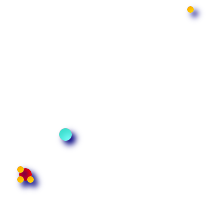# ST_GeometricMedian

## Name

ST_GeometricMedian — Returns the geometric median of a MultiPoint.

## Synopsis

``` geometry ST_GeometricMedian ( ``` geometry g , float8 tolerance , int max_iter , boolean fail_if_not_converged ``` ) ``` ;

## Description

Computes the approximate geometric median of a MultiPoint geometry using the Weiszfeld algorithm. The geometric median provides a centrality measure that is less sensitive to outlier points than the centroid. The algorithm will iterate until the distance change between successive iterations is less than the supplied ``` tolerance ``` parameter. If this condition has not been met after ``` max_iterations ``` iterations, the function will produce an error and exit, unless ``` fail_if_not_converged ``` is set to false. If a tolerance value is not provided, a default tolerance value will be calculated based on the extent of the input geometry.

Availability: 2.3.0This function supports 3d and will not drop the z-index.

## ExamplesComparison of the centroid (turquoise point) and geometric median (red point) of a four-point MultiPoint (yellow points).

```WITH test AS (
SELECT 'MULTIPOINT((0 0), (1 1), (2 2), (200 200))'::geometry geom)
SELECT
ST_AsText(ST_Centroid(geom)) centroid,
ST_AsText(ST_GeometricMedian(geom)) median
FROM test;
centroid      |                 median
--------------------+----------------------------------------
POINT(50.75 50.75) | POINT(1.9761550281255 1.9761550281255)
(1 row)
```